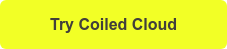# Storing Dask DataFrames in Memory with persist

December 14, 2021

You can store DataFrames in memory with Dask persist which will make downstream queries that depend on the persisted data faster.  This is great when you perform some expensive computations and want to save the results in memory so they’re not rerun multiple times.

Many Dask users erroneously assume that Dask DataFrames are persisted in memory by default, which isn’t true.  Dask runs computations in memory.  It doesn’t store data in memory unless you explicitly call `persist()`.

This post will teach you about when to persist DataFrames and the best practices.  You’ll see some examples that demonstrate how to take queries that take more than a minute to execute normally to run in less than a second once the data is persisted in memory.

Let’s start with examples on small DataFrames so you can see the syntax and then on larger DataFrames so you can see some performance benchmarks.

## Simple example with Dask persist

Let’s create a small Dask DataFrame to demonstrate how Dask persist works on DataFrames.

```import dask.dataframe as dd
import pandas as pd

df = pd.DataFrame({"col1": ["a", "b", "c", "d"], "col2": [1, 2, 3, 4]})
ddf = dd.from_pandas(df, npartitions=2)

persisted_ddf = ddf.persist()

len(persisted_ddf)```

The persisted_ddf is saved in memory when `persist()` is called.  Subsequent queries that run off of` persisted_ddf` will execute quickly.

Let’s run `persist()` on a larger DataFrame to see some real computation runtimes.

## Dask persist on big dataset

Let’s run some queries on a dataset that’s not persisted to get some baseline query runtimes.  Then let’s persist the Dask DataFrame, run the same queries, and quantify the performance gains from persisting the dataset.

These queries are run on a 662 million row dataset.  Here are 5 rows of sample data.

Here’s the code that creates a computation cluster, reads in a DataFrame, and then creates a filtered DataFrame.

```import coiled

cluster = coiled.Cluster(name="powers", n_workers=5)

"s3://coiled-datasets/timeseries/20-years/parquet",
storage_options={"anon": True, "use_ssl": True},
engine="pyarrow",
)```

Let’s time a couple of analytical queries.

```res = ddf.loc[ddf["id"] > 1150]

len(res) # 87 seconds

res.name.nunique().compute() # 62 seconds```

Let’s persist the Dask DataFrame and then run the same queries to see how long they take to execute.

```persisted_res = res.persist()

len(persisted_res) # 2 seconds

persisted_res.name.nunique().compute() # 2 seconds```

The queries took over a minute to run before the data was persisted, but only take 2 seconds to run on the persisted dataset.

Of course, it takes some time to persist the data.  Let’s look at why this particular example gave us great results when persisting Dask DataFrames.

## Great opportunity to run Dask persist

Persisting helps sometimes and causes problems other times.  Let’s look at high-level patterns when it’ll usually help and when it’ll usually cause problems.

Our example uses the following pattern:

• Filter it down to a much smaller datasets (that’s much smaller than the memory of the cluster)
• Run analytical queries on the filtered dataset

You can expect good results from Dask `persist()` with this set of circumstances.

Here’s a different pattern that won’t usually give such a good result.

• Read in a large dataset that’s bigger than memory
• Persist the entire dataset
• Run a single analytical operation

In this case, the cost of running the persist operation will be greater than the benefits of having a single query run a little bit faster.  Persisting doesn’t always help.

## Writing to disk vs persisting Dask DataFrame

We can also “persist” results by writing to disk rather than saving the data in memory.

Let’s write the filtered dataset in S3 and run the analytical queries to quantify time savings.

```res.repartition(2).to_parquet(
"s3://coiled-datasets/tmp/matt/disk-persist", engine="pyarrow"
)

"s3://coiled-datasets/tmp/matt/disk-persist",
storage_options={"anon": True, "use_ssl": True},
engine="pyarrow",
)

len(df) # 0.4 seconds

df.name.nunique().compute() # 0.3 seconds```

The filtered dataset that was written to disk can be queried with subsecond response times.

Writing temporary files to disk isn’t always ideal because then you have stale files sitting around that need to get cleaned up later.

## Repartitioning and then using Dask persist

We can also repartition before persisting Dask DataFrames, which will make our analytical queries in this example run even faster.

```res2 = res.repartition(2)

persisted_res2 = res2.persist()

len(persisted_res2) # 0.3 seconds

persisted_res2.name.nunique().compute() # 0.3 seconds```

The filtered dataset is tiny and doesn’t need a lot of partitions.  That’s why repartitioning drops query times from around 2 seconds to 0.3 seconds in this example.

## Compute vs Persist Dask DataFrame

Compute and persist are both ways to materialize results in memory.

Compute materializes results in a pandas DataFrame and Dask persist materializes the results in a Dask DataFrame.

Compute only works for tiny datasets that fit in the memory of a single machine.  Larger results can be persisted because the data can be spread in the memory of multiple computers in a cluster.

See this blog post on compute() for more details.

It’s especially useful when you’ve performed some expensive operations that reduce the dataset size and subsequent operations benefit from having the computations stored.

Some new programmers can misuse Dask persist and slow down analyses by persisting Dask DataFrames too often or trying to persist massive datasets.  It helps sometimes, as shown in this blog post, but it can cause analyses to run slower when used incorrectly.

Persisting Dask DataFrames will generally speed up analyses when these sets of facts are true:

• You’ve performed expensive computations that have reduced the dataset size
• The reduced dataset comfortably fits in memory
• You want to run multiple queries on the reduced dataset

Thanks for reading! And if you’re interested in trying out Coiled Cloud, which provides hosted Dask clusters, docker-less managed software, and one-click deployments, you can do so for free today when you click below.